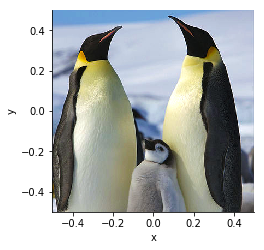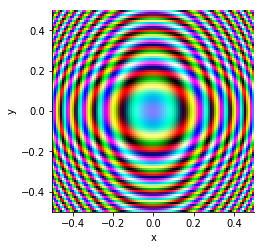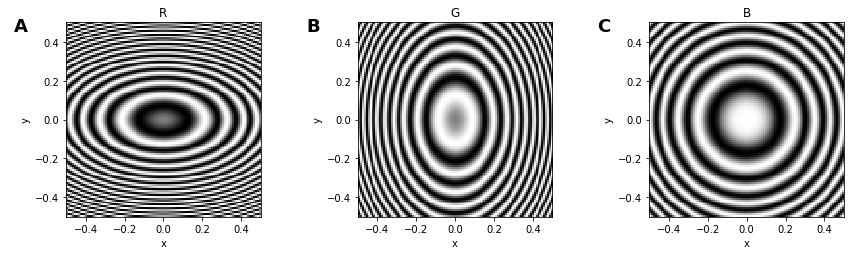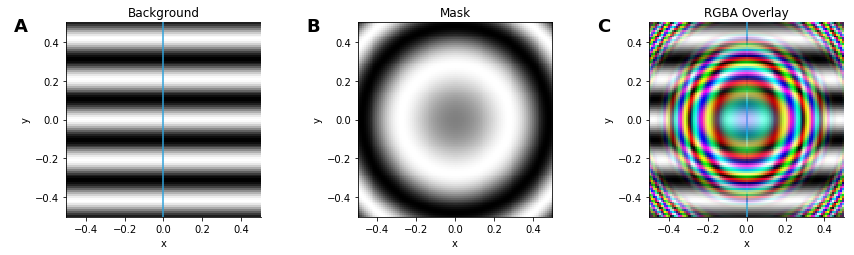# Rgb¶

Title
RGB Element
Dependencies
Matplotlib
Backends
Matplotlib
Bokeh
In :
import numpy as np
import holoviews as hv
from holoviews import opts

hv.extension('matplotlib')RGB represents a regularly spaced 2D grid of an underlying continuous space of RGB(A) (red, green, blue and alpha) color space values. The definition of the grid closely matches the semantics of an Image and in the simplest case the grid may be specified as a NxMx3 or NxMx4 array of values along with a bounds, but it may also be defined through explicit and regularly spaced x/y-coordinate arrays. The two most basic supported constructors of an RGB element therefore include:

RGB((X, Y, R, G, B))



where X is a 1D array of shape M, Y is a 1D array of shape N and R/G/B are 2D array of shape NxM, or equivalently:

RGB(Z, bounds=(x0, y0, x1, y1))



where Z is a 3D array of stacked R/G/B arrays with shape NxMx3/4 and the bounds define the (left, bottom, right, top) edges of the four corners of the grid. Other gridded formats which support declaring of explicit x/y-coordinate arrays such as xarray are also supported. See the Gridded Datasets user guide for all the other accepted data formats.

One of the simplest ways of creating an RGB element is to load an image file (such as PNG) off disk, using the load_image classmethod:

In :
hv.RGB.load_image('../assets/penguins.png')

Out:If you have PIL or pillow installed, you can also pass in a PIL Image as long as you convert it to Numpy arrays first:

from PIL import Image
hv.RGB(np.array(Image.open('../assets/penguins.png')))

This Numpy-based method for constructing an RGB can be used to stack up arbitrary 2D arrays into a color image:

In :
x,y = np.mgrid[-50:51, -50:51] * 0.1

r = 0.5*np.sin(np.pi  +3*x**2+y**2)+0.5
g = 0.5*np.sin(x**2+2*y**2)+0.5
b = 0.5*np.sin(np.pi/2+x**2+y**2)+0.5

hv.RGB(np.dstack([r,g,b]))

Out:You can see how the RGB object is created from the original channels:

In :
opts.defaults(opts.Image(cmap='gray'))

hv.Image(r,label="R") + hv.Image(g,label="G") + hv.Image(b,label="B")

Out:RGB also supports an optional alpha channel, which will be used as a mask revealing or hiding any Elements it is overlaid on top of:

In :
mask = 0.5*np.sin(0.2*(x**2+y**2))+0.5

bg = hv.Image(0.5*np.cos(x*3)+0.5, label="Background") * hv.VLine(x=0,label="Background")
overlay = (bg*rgba).relabel("RGBA Overlay")For full documentation and the available style and plot options, use hv.help(hv.RGB).